# Solvability of reactions in rigid multibody systems with redundant nonholonomic constraints

## Abstract

The problem of calculating joint reaction forces in rigid body mechanisms with redundant constraints, both geometric and nonholonomic, is discussed. When constraint equations are dependent, some of the constraint reactions are unsolvable, i.e., cannot be uniquely determined using a rigid body model, whereas some others may be solvable. In this paper, analytic conditions, which must be fulfilled to obtain unique values of selected reaction forces in the presence of dependent nonholonomic constraints, are presented and proven. The concept of direct sum, known from linear algebra, is exploited. These purely mathematical conditions are followed by numerical methods that enable detection of constraints with uniquely solvable reactions. Similar conditions and methods were proposed earlier for holonomic systems. In this contribution, they are generalized to the case of linear nonholonomic constraints. An example of constraint reactions solvability analysis, for a mechanism subjected to redundant nonholonomic constraints, is presented.

## Introduction

Dynamic analysis of a multibody system consists in calculating motion resulting from loads and driving constraints imposed on the system [10, 16, 28]. The time history of kinematic quantities, i.e., positions, velocities, and accelerations of bodies, is determined during the analysis. It is not necessary to calculate constraint reaction forces when the system is frictionless and its motion is the only object of interests . Nevertheless, constraint reactions are frequently calculated along with the kinematic parameters describing motion, since they are needed, e.g., for design purposes or structural analyses.

In multibody modeling, the constraint reactions not always can be uniquely determined. The problem of joint reactions indeterminacy, in engineering simulations of rigid multibody systems, is most often caused by redundant constraints presence. Redundant constraints are defined as constraints that can be removed without changing the kinematics of the system, i.e., they appear when the same degree of freedom is restricted more than once. Real-world mechanisms quite often are purposely overconstrained, mainly in order to strengthen or simplify their construction. Redundant constraints can be present in both holonomic and nonholonomic systems.

Multibody modeling of overconstrained systems is impeded. When redundant constraints are present in the mathematical model of holonomic multibody system, the constraint equations are dependent [10, 16]; hence, the constraint Jacobian matrix is rank-deficient. Therefore, special approaches are needed to handle equations of motion, e.g., [15, 20, 23, 27].

In the context of this article subject, special attention should be paid to the joint reactions calculation. In multibody modeling, sometimes it is assumed that constraint equations are independent (e.g., [4, 19]), hence redundant constraints, and thus reactions solvability problems, are simply ignored. Most often, however, redundant constraints are handled using one of two essentially different approaches. The first one consists in modifying the set of equations in order to exclude dependent equations [16, 28] (redundant constraints elimination is an example of this approach). The second one consists in leaving the set of equations unchanged and solving it using algorithms capable to deal with dependent equations (the minimum norm solution, sometimes based on the pseudoinverse technique , and the augmented Lagrangian formulation [2, 3] can serve as examples of this approach). In either case, purely mathematical operations, not supported by laws of physics, are performed to find the reactions . Hence, the obtained solution usually reflects properties of the redundant constraints handling method rather than physical properties of the investigated multibody system. It should be noted, however, that contribution  indicates that in some cases combination of additional weighting factors (which are tuned to reflect elasticity of links and joints) with minimum norm solution leads to calculation of realistic reactions. So far, no general method of factors selection is proposed. Similarly, in the case of penalty methods, the authors of [13, 14, 30] formulated an idea that a flexibility aspect can be incorporated by relating the penalty factors to the real mechanism stiffness, which allows us to solve the indeterminacy problem without adding any major complication to equations of motion. However, so far no general rules on how to relate the penalty parameters with flexibility properties of multibody system parts and joints are provided.

A direct consequence of constraints redundancy is that some or all constraint reactions cannot be uniquely determined using a rigid body model . Moreover, in the case of Coulomb friction in joints, the simulated motion might be nonunique . It can be proven, however, that in the case of an overconstrained rigid body mechanism subjected to holonomic constraints, despite the fact that all constraint reactions cannot be uniquely determined, selected reactions or selected groups of reactions can be specified uniquely [33, 34]. In , methods of constraint reaction solvability analysis were proposed. An algebraic criterion, allowing detection of the joints for which reactions are uniquely solvable, was formulated. This criterion was followed by numerical methods for finding such joints.

In this contribution, systems subjected to both holonomic and linear nonholonomic constraints are investigated. It is shown that when redundancy problems and reaction forces are concerned, both types of constraints can be treated jointly and uniformly. The methods of constraint reactions solvability analysis are generalized to systems subjected to linear nonholonomic constraints. Firstly, the algebraic criterion is revisited and its applicability to nonholonomic systems is considered. Then numerical methods of reactions solvability analysis are discussed. Finally, an exemplary system with redundant nonholonomic constraints is analyzed.

## Equations of motion for constrained systems

### Generalized coordinates and constraint equations

Consider a rigid multibody system described by n dependent generalized coordinates q. Holonomic constraints imposed on coordinates are represented by a set of m nonlinear scalar algebraic equations:

$$\boldsymbol{\varPhi} ( \mathbf{q},t ) = \mathbf{0}_{m \times 1}.$$
(1)

We assume that constraints (1) are consistent, i.e., no contradictory conditions are imposed on coordinates q.

The holonomic constraint equations (1) can be differentiated with respect to time to obtain constraints for velocities:

$$\boldsymbol{\varPhi}_{\mathbf{q}}\dot{\mathbf{q}} + \boldsymbol{ \varPhi}_{t} = \mathbf{0}_{m \times 1},$$
(2)

where Φ q is the constraint Jacobian matrix which may be rank-deficient and Φ t is the vector of partial derivatives with respect to time.

Linear nonholonomic and scleronomic constraints (Pfaffian constraints) are represented by a set of h scalar equations, linear in velocities $$\dot{\mathbf{q}}$$ :

$$\boldsymbol{\varPsi} ( \mathbf{q} )\dot{\mathbf{q}} = \mathbf{0}_{h \times 1}.$$
(3)

Note that in the case of redundant constraints presence, matrix Ψ can be rank-deficient.

It should be emphasized that the existence of a single nonintegrable velocity constraint does not necessarily mean that a system is nonholonomic, since this constraint may prove to be integrable by virtue of the remaining constraint equations. To check the holonomy of the system, the Frobenius theorem may be employed [5, 26]. It is important that our further considerations are valid for both integrable and nonintegrable Pfaffian constraints.

Equation (3) can be appended to Eq. (2) to obtain velocity level constraints for the whole system:

$$\mathbf{C}\dot{\mathbf{q}} + \mathbf{b} = \mathbf{0},$$
(4)

where respective definitions of coefficient matrix C (often called a constraint matrix) and vector b are the following:

$$\mathbf{C}(\mathbf{q},t ) = \left [ \begin{array}{c} \boldsymbol{\varPhi}_{\mathbf{q}} \\ \boldsymbol{\varPsi} \end{array} \right ]_{(m + h) \times n}, \qquad \mathbf{b}(\mathbf{q},t ) = \left [ \begin{array}{c} \boldsymbol{\varPhi}_{t} \\ \mathbf{0} \end{array} \right ].$$
(5)

We assume that constraints (4) are consistent, i.e., no contradictory conditions are imposed on velocities $$\dot{\mathbf{q}}$$. For our further considerations, it is important that when the mechanism is overconstrained, rows of matrix C are linearly dependent, hence

$$r = \mathrm{rank}( \mathbf{C} ) < m + h.$$
(6)

It is worth noting that constraint matrix C may be rank-deficient even when both Φ q and Ψ are full-rank matrices.

The degree of redundancy p is defined as

$$p = m + h - r.$$
(7)

The velocity constraints (4) can be differentiated with respect to time in order to obtain constraints for accelerations:

$$\mathbf{C}\ddot{\mathbf{q}} - \boldsymbol{\varGamma} = \mathbf{0}_{(m + h) \times 1},$$
(8)

with vector Γ defined as

$$\boldsymbol{\varGamma} = - \left [ \begin{array}{c} ( \boldsymbol{\varPhi}_{\mathbf{q}}\dot{\mathbf{q}} )_{\mathbf{q}}\dot{\mathbf{q}} + 2\boldsymbol{\varPhi}_{t\mathbf{q}}\dot{\mathbf{q}} + \boldsymbol{\varPhi}_{tt} \\ ( \boldsymbol{\varPsi}\dot{\mathbf{q}})_{\mathbf{q}}\dot{\mathbf{q}} \end{array} \right ].$$
(9)

In our further considerations, we will utilize absolute Cartesian coordinates [16, 28]. The main reason for choosing this type of coordinates is the fact that, in this approach, all kinematic pairs are treated in the same way, i.e., each pair is represented as a subset of Eq. (4). In the case of other types of coordinates, e.g., relative joint coordinates, the constraint equations are formulated only for selected, loop closing joints. The uniform treatment of all kinematic pairs simplifies calculation of joint reaction forces, however, it should be noted that the problem of joint reaction solvability—crucial for our considerations—is an issue of mechanism’s structure, and from this point of view the choice of coordinates is irrelevant.

### Equations of motion

Equations of motion for the considered multibody system can be written in the following form [10, 16]:

$$\mathbf{M}\ddot{\mathbf{q}} + \mathbf{C}^{T}\boldsymbol{ \lambda} = \mathbf{F}( \mathbf{q},\dot{\mathbf{q}},t ),$$
(10)

where M denotes the mass matrix (which is invertible), F is the vector containing the external forces as well as the velocity dependent inertia terms, and λ is the vector of Lagrange multipliers.

The Lagrange equations of the first kind (Eq. (10)) and the acceleration level constraints (Eq. (8)) can be written jointly in a matrix form, to obtain an index-1 set of differential-algebraic equations:

$$\left [\begin{array}{c@{\quad }c} \mathbf{M} & \mathbf{C}^{T} \\ \mathbf{C} & \mathbf{0} \end{array} \right ] \left [ \begin{array}{c} \ddot{\mathbf{q}} \\ \boldsymbol{\lambda} \end{array} \right ]= \left [ \begin{array}{c} \mathbf{F} \\ \boldsymbol{\varGamma} \end{array} \right ].$$
(11)

Equation (11) can be solved for the accelerations and the Lagrange multipliers (it can be shown that the Lagrange multipliers λ are uniquely solvable only when matrix CM −1 C T is nonsingular, i.e., when matrix C has full row rank). In the case of constraints redundancy, however, matrix C is rank deficient, thus the leading matrix in Eq. (11) becomes singular, and Eq. (11) has a nonunique solution. More precisely, the solution for accelerations $$\ddot{\mathbf{q}}$$ is unique, and the solution for multipliers λ is non-unique. To justify this statement, it is helpful to define matrix V n×(nr), which is an orthogonal complement to C (which is now assumed to be rank deficient, as indicated in Eq. (6)), so that

$$\mathbf{C}\mathbf{V} = \mathbf{0}\quad \mathrm{and}\quad \mathrm{rank}\left ( \left [ \begin{array}{c} \mathbf{V}^{T} \\ \mathbf{C} \end{array} \right ]_{(n + p) \times n} \right ) = n.$$
(12)

Premultiplying the first equation of Eq. (11) by V T eliminates the Lagrange multipliers (it is very important that F does not depend on λ, hence λ does not appear on the right-hand side of Eq. (11) and can be eliminated) and yields the following set of equations (sometimes called a null space formulation [18, 29]):

$$\left [\begin{array}{c} \mathbf{V}^{T}\mathbf{M} \\ \mathbf{C} \end{array} \right ]\ddot{\mathbf{q}} = \left [ \begin{array}{c} \mathbf{V}^{T}\mathbf{F} \\ \boldsymbol{\varGamma} \end{array} \right ].$$
(13)

Matrix M is invertible, thus—due to Eq. (12)—the rank of the leading matrix in Eq. (13) equals n. Constraints (8) are consistent; hence Eq. (13) has a unique solution for $$\ddot{\mathbf{q}}$$. Obviously, it is possible to find another matrix V V, spanning the null space of the constraint matrix C, and to obtain a different version of Eq. (13). In such a case, the following holds:

$$\mathbf{V}^{*} = \mathbf{V}\mathbf{A}_{(n - r) \times (n - r)},$$
(14)

where A is an invertible transformation matrix. Thus, when V appears instead of V in Eq. (13), it is sufficient to premultiply the first equation by A T, to obtain the original form of Eq. (13). This indicates that the solution for $$\ddot{\mathbf{q}}$$ does not depend on the choice of matrix V, thus it is a unique solution.

It should be noted that, since only constraints at acceleration level are represented in Eq. (11), some stabilization techniques are usually adopted to account for constraints given by Eqs. (1) and (4).

## Constraint reactions

### Methods of handling redundant constraints

The presence of redundant constraints induces rank deficiency of constraint matrix C, thus special methods are needed to handle equations of motion. It is possible to use algorithms capable to deal with dependent equations, e.g., the pseudoinverse-based methods  or the augmented Lagrangian method [2, 3]. The other possibility is to modify the set of equations in order to exclude dependent equations [16, 28]; and this approach—usually referred to as redundant constraints elimination method—was adopted here to simulate the exemplary mechanism, presented in the subsequent section. It should be emphasized that, regardless of the method used to handle redundant constraints, the problems with Lagrange multipliers uniqueness are circumvented, not solved .

The redundant constraints elimination method consists in removing dependent equations from the mathematical description of multibody system, hence only the subset of independent equations is analyzed. The redundant constraints elimination may be done “manually”—selected constraints, which are unnecessary when kinematics is concerned, are simply not included into the model . The other possibility is to eliminate redundant constraints automatically. The automatic elimination may be performed prior to constraint equations formulation  or during the preprocessing phase of simulation . However, the most popular and the simplest method of automatic redundant constraints elimination (implemented in widely used multibody software) is based on the constraint matrix C investigation [16, 28]. In this method, all constraint equations are formulated and then they are divided into subsets of independent and dependent equations. The division is usually made according to the results of the constraint matrix decomposition. The equations classified as dependent are excluded from the mathematical model.

It must be stressed that, regardless of redundant constraints selection method, the reactions associated with eliminated constraints are set to zero. It is quite obvious that in real mechanisms reactions corresponding to constraints neglected during analysis are not likely to be constantly equal zero. Moreover, it is worth noting that setting the reactions of eliminated constraints to zero, transfers their loads to the constraints that remain in the mathematical model. Consequently, redundant constraints elimination affects not only reactions of excluded constraints but also reactions of remaining constraints.

### Uniquely determined constraint reactions

The generalized constraint reactions, for the whole multibody system, can be calculated as [10, 16, 28]:

$$\mathbf{f} = \mathbf{C}^{T}\boldsymbol{\lambda} = \mathbf{F} - \mathbf{M}\ddot{\mathbf{q}}.$$
(15)

Similarly, the generalized reactions in a specified kinematic pair can be calculated as

$$\mathbf{f}_{X} = \mathbf{C}_{X}^{T} \boldsymbol{\lambda}_{X},$$
(16)

where C X denotes a matrix built of these rows of C that correspond to constraint equations representing the kinematic pair, and λ X is a vector of Lagrange multipliers associated with these constraints. Note that Eq. (4) can always be reordered, so that

$$\mathbf{C} = \left [ \begin{array}{c} \mathbf{C}_{X} \\ \mathbf{C}_{Y} \end{array} \right ],$$
(17)

where none of the rows of matrix C Y corresponds to the considered kinematic pair.

In the case of redundant constraints presence, the generalized reactions for the whole system (f) are uniquely determined, however, some or all reactions between pairs of interacting bodies (f X ) cannot be uniquely determined using a rigid body model. In other words, it is impossible to tell how the total reaction f is distributed between the individual constraint reactions f X . This is an immediate consequence of the nonuniqueness of multipliers λ, discussed in Sect. 2.2.

Fortunately, in many cases, some of the constraint reactions are solvable (i.e., they can be uniquely determined using a rigid body model) despite the presence of redundant constraints in the system taken as a whole. It is possible to generalize the methods of reactions solvability analysis, presented in  (for holonomic systems only), to the case of systems subjected to both holonomic and linear nonholonomic constraints.

The concept of direct sum, known from linear algebra , can be exploited to check whether the particular examined reactions can be uniquely calculated. To recall the definition of direct sum, assume that Z is a linear vector space in R n whereas X and Y are the subspaces of Z. We say that Z is a direct sum of subspaces X and Y, and we denote it as Z=XY, when the following conditions are fulfilled:

1. 1.

Z is a sum of subspaces X and Y (we denote it as Z=X+Y), which means that any vector zZ can be represented as z=x+y, where xX and yY.

2. 2.

If x 1+y 1=x 2+y 2, provided that x 1, x 2X, and y 1, y 2Y, then x 1=x 2 and y 1=y 2.

To formulate analytical condition for checking constraint reactions solvability, let us consider a kinematic pair represented by holonomic or nonholonomic constraint equations. Let X be a linear space spanned by these columns of matrix C T that correspond to the constraints representing this kinematic pair $$(X = \mathrm{span} (\mathbf{C}_{X}^{T}))$$, and let Y be a linear space spanned by the other columns of matrix C T $$(Y = \mathrm{span}( \mathbf{C}_{Y}^{T} ))$$. Moreover, let Z be a linear space spanned by all columns of matrix C T (Z=span(C T)). Note that in the case of constraint redundancy, the following inequality is valid (compare Eq. (6)):

$$\mathrm{dim}( Z ) = \mathrm{rank}( \mathbf{C} ) = r < m + h,$$
(18)

thus the same vector of generalized reactions f can be obtained for different vectors of Lagrange multipliers λ (see Eq. (15)).

It can be shown that:

If Z is a direct sum of X and Y (Z=XY), then the generalized constraint reaction f X in the considered kinematic pair is uniquely determined.

To prove the above statement, the properties of direct sum can be utilized. Let us write the Lagrange multipliers vector as $$\boldsymbol{\lambda} = [\begin{array}{c@{\ }c} \boldsymbol{\lambda}_{X}^{T} & \boldsymbol{\lambda}_{Y}^{T} \end{array} ]^{T}$$, where λ X corresponds to the vectors spanning the linear space X and λ Y corresponds to the vectors spanning the linear space Y. Assume that λ and λ ∗∗ are two different vectors of Lagrange multipliers. The generalized reactions corresponding to these multipliers are the following:

$$\mathbf{f}^{*} = \mathbf{C}^{T}\boldsymbol{ \lambda}^{*} = \mathbf{C}_{X}^{T}\boldsymbol{ \lambda}_{X}^{*} + \mathbf{C}_{Y}^{T} \boldsymbol{\lambda}_{Y}^{*} = \mathbf{f}_{X}^{*} + \mathbf{f}_{Y}^{*}$$
(19)

and

$$\mathbf{f}^{**} = \mathbf{C}^{T}\boldsymbol{ \lambda}^{**} = \mathbf{C}_{X}^{T}\boldsymbol{ \lambda}_{X}^{**} + \mathbf{C}_{Y}^{T} \boldsymbol{\lambda}_{Y}^{**} = \mathbf{f}_{X}^{**} + \mathbf{f}_{Y}^{**}.$$
(20)

Since f =f ∗∗, the 2nd condition of the direct sum definition yields $$\mathbf{f}_{X}^{*} = \mathbf{f}_{X}^{**}$$ (and similarly, $$\mathbf{f}_{Y}^{*} = \mathbf{f}_{Y}^{**}$$).

The presented considerations show that, when the direct sum condition is fulfilled, the term $$\mathbf{f}_{X} = \mathbf{C}_{X}^{T}\boldsymbol{\lambda}_{X}$$ (generalized reaction force in the considered kinematic pair—see Eq. (16)) can be uniquely determined. It should be stressed, however, that the problem of finding Lagrange multipliers λ X may not have a unique solution. The Lagrange multipliers λ X can be uniquely determined only if C X is a full rank matrix.

The above results can be utilized to check whether constraint reactions in the selected kinematic pair can be uniquely determined, regardless of possibility that the problem of calculating Lagrange multipliers may have infinitely many solutions.

### Numerical detection of uniquely determined constraint reactions

Three numerical methods of examination whether the direct sum condition is fulfilled, and thus whether reactions in the particular kinematic pair are uniquely solvable, were proposed in . The first method is based on ranks comparison of matrices C X , C Y , and C, the second is based on singular value decomposition of matrix C; these methods will not be discussed here. This paper presents an improved version of the third method (the most effective one), which is based on QR decomposition of the constraint matrix C.

The QR method decomposes matrix C into matrices [12, 17]:

$$\mathbf{C}\mathbf{E} = \mathbf{Q}\mathbf{R} \quad \mbox{or}\quad \mathbf{C}^{T} = \mathbf{E}\mathbf{R}^{T}\mathbf{Q}^{T},$$
(21)

where Q is an orthogonal ((m+h)×(m+h)) matrix, E is an orthogonal (n×n) permutation matrix (it consists of zeros and ones only) and R is a rectangular ((m+hn) upper triangular matrix.

In the literature, one can easily find a QR decomposition without the use of permutation matrix E, however, this variant of decomposition is not convenient for us. The column permutation E is chosen in such a way that absolute values of diagonal elements in R are decreasing. This fact is important for our considerations, since it assures that, for decomposed matrix C with rank r, the last p (see Eq. (7)) rows of matrix R consist of zeros only.

The QR decomposition of constraint matrix C is useful when formulating a numerical method of checking whether an arbitrarily chosen vector wZ can be decomposed into sum of two vectors w X X and w Y Y, both of them given uniquely. The proposed criterion is based on the 2nd condition of direct sum of spaces definition.

Let us consider vector wZ and write it as a linear combination of matrix C T columns:

$$\mathbf{w} = \mathbf{w}_{X} + \mathbf{w}_{Y} = \mathbf{C}_{X}^{T}\mathbf{n}_{X} + \mathbf{C}_{Y}^{T}\mathbf{n}_{Y} = \mathbf{C}^{T}\mathbf{n} = \mathbf{E}\mathbf{R}^{T} \mathbf{Q}^{T}\mathbf{n},$$
(22)

where $$\mathbf{n}_{(m + h) \times 1} = [ \begin{array}{c@{\ }c} \mathbf{n}_{X}^{T} & \mathbf{n}_{Y}^{T} \\ \end{array} ]^{T}$$ is a vector of linear combination coefficients.

An auxiliary vector d, defined below, is more suitable for further considerations:

$$\mathbf{d}_{(m + h) \times 1} = \mathbf{Q}^{T}\mathbf{n}.$$
(23)

The transformation (23) maps d to n and n to d uniquely, since Q is a square and orthogonal matrix. This transformation can be used to rewrite Eq. (22) in the following form:

$$\mathbf{w} = \mathbf{C}^{T}\mathbf{n} = \mathbf{E} \mathbf{R}^{T}\mathbf{d}.$$
(24)

We can state that Eq. (24) uniquely defines only the first r elements of vector d, since only the first r rows of matrix R are nonzero. The remaining p elements of d can be arbitrarily chosen. Vector d satisfying Eq. (24), and thus vector n, can be written in the following form: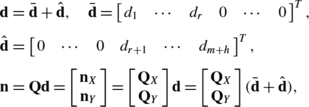(25)

where elements d 1,…,d r are uniquely defined by (24) and elements d r+1,…,d m+h can be arbitrarily chosen; Q X denotes a matrix consisting of only these rows of Q that correspond to the considered kinematic pair.

Hence, vector w X can be written in the form:

$$\mathbf{w}_{X} = \mathbf{C}_{X}^{T} \mathbf{n}_{X} = \mathbf{C}_{X}^{T} \mathbf{Q}_{X}\bar{\mathbf{d}} + \mathbf{C}_{X}^{T} \mathbf{Q}_{X}\hat{\mathbf{d}}.$$
(26)

The first r elements of vector $$\hat{\mathbf{d}}$$ are zeros, the remaining p can be chosen arbitrarily. Thus, we can state that vector w X can be determined uniquely only when the arbitrary elements of $$\hat{\mathbf{d}}$$ vanish due to multiplication by zero. This observation implies the following definition of an auxiliary matrix B X :

$$[ \mathbf{B}_{X} ]_{n \times p} = \mathbf{C}_{X}^{T} \mathbf{Q}_{X}^{\mathrm{col}},$$
(27)

where matrix $$\mathbf{Q}_{X}^{\mathrm{col}}$$ consists of last p columns of Q X .

In conclusion, we can say that vector w X can be determined uniquely when matrix B X consists of zeros only. This result can be easily used for detecting kinematic pairs for which reactions can be uniquely determined. Firstly, the QR decomposition of the constraints matrix C should be calculated. Then, rows of matrices Q and C, corresponding to the kinematic pair being investigated, ought to be extracted. Finally, matrix B X should be calculated and examined if it contains only zero elements.

It should be mentioned that the method presented here, as well as two other methods described in , merely require operations on the constraint matrix C, therefore, it is relatively easy to implement them in a multibody code.

## Example

### Structure and kinematics of the mechanism

Since applications of multibody methods to robotics have always been interesting to us [7, 9, 22], a mobile robot was a natural candidate to serve as an example in this paper. The constraint reaction solvability method was utilized to analyze a simplified model of a four-wheel mobile robot connected with a one-wheel trolley (Fig. 1). The system is modeled as a planar mechanism, in which wheels are replaced by semicircular knife edges. Edges W 1 and W 2 can change their inclinations with respect to the robot platform 1, whereas edges W 3 and W 4 are rigidly attached to the platform. The linkage consisting of bodies 2, 3, 4, 5, and 6, driven in joint H, is used to rotate edges W 1 and W 2 in a synchronized manner. Due to appropriately chosen linkage dimensions, it is possible to change the wheel inclination by ±90°. The edge W 5 is rigidly attached to the trolley platform 7. The trolley is connected to the robot via revolute joint A.

A global inertial reference frame π 0=x 0 y 0 is established on the ground 0, and body-fixed local reference frames π i =x i y i are embedded in the moving bodies (Fig. 1). The absolute Cartesian coordinates that describe the mechanism form vector q:

$$\mathbf{q} = \left [ \begin{array}{c@{\quad }c@{\quad }c@{\quad }c@{\quad }c@{\quad }c@{\quad }c} \mathbf{q}_{1}^{T} & \mathbf{q}_{2}^{T} & \mathbf{q}_{3}^{T} & \mathbf{q}_{4}^{T} & \mathbf{q}_{5}^{T} & \mathbf{q}_{6}^{T} & \mathbf{q}_{7}^{T} \end{array} \right ]^{T},\qquad \mathbf{q}_{i} = \left [ \begin{array}{c@{\quad }c} \mathbf{r}_{i}^{T} & \varphi_{i} \end{array} \right ]^{T},$$
(28)

where $$\mathbf{r}_{i} = [ \begin{array}{c@{\ }c} x_{i} & y_{i} \\ \end{array} ]^{T}$$ represents the position of the local reference frame π i origin with respect to the global frame π 0, and φ i is the angle of the local frame π i rotation with respect to the global frame π 0.

Seven revolute joints (A, B, C, D, E, F, G) and one translational joint (H) are described by holonomic (geometric) constraints. Appropriate equations are presented in the Appendix. Driving constraints (represented by holonomic and rheonomic constraints, described in the Appendix) and are imposed on relative motion in joint H. The time history of the displacement d(t) of body 6 with respect to body 1 is presented in Fig. 2. The steering is chosen so that the robot, which initially is moving along x 0 axis, firstly turns right, then travels forward, and finally turns left and starts to move along a line parallel to x 0.

The holonomic constraints imposed on the modeled multibody system can be written jointly as a set of m=17 nonlinear algebraic equations:

$$\boldsymbol{\varPhi} ( \mathbf{q},t ) = \left [ \begin{array}{c@{\quad }c@{\quad }c@{\quad }c@{\quad }c@{\quad }c@{\quad }c@{\quad }c@{\quad }c} \boldsymbol{\varPhi}_{A}^{r^T} & \boldsymbol{\varPhi}_{B}^{r^T} & \boldsymbol{\varPhi}_{C}^{r^T} & \boldsymbol{\varPhi}_{D}^{r^T} & \boldsymbol{\varPhi}_{E}^{r^T} & \boldsymbol{\varPhi}_{F}^{r^T} & \boldsymbol{\varPhi}_{G}^{r^T} & \boldsymbol{\varPhi}_{H}^{t^T} & \varPhi_{H}^{d} \end{array} \right ]^{T} = \mathbf{0}_{17 \times 1}.$$
(29)

Formulas for the constraint Jacobian matrix Φ q and for vector Φ t (see Eq. (2)) as well as for Γ vector (see Eq. (9)) are presented in the Appendix.

The ground-wheel interactions are represented by knife-edge Pfaffian constraints. The knife-edge constraint equation can be derived by requiring that linear velocity at the point of contact is parallel to the edge. The following scalar equation, linear in velocities, is obtained:

$$\mathbf{n}_{K}^{(0)^T}\mathbf{v}_{K}^{(0)} = \bigl( \mathbf{R}_{i}\mathbf{n}_{K}^{(i)} \bigr)^{T} \bigl(\dot{\mathbf{r}}_{i} + \boldsymbol{\varOmega} \mathbf{R}_{i}\mathbf{s}_{K}^{(i)}\dot{ \varphi}_{i} \bigr) = \left [ \begin{array}{c@{\quad }c} ( \mathbf{R}_{i}\mathbf{n}_{K}^{(i)})^{T} & \mathbf{n}_{K}^{(i)^T}\boldsymbol{\varOmega} \mathbf{s}_{K}^{(i)} \end{array} \right ]\left [ \begin{array}{c} \dot{\mathbf{r}}_{i} \\ \dot{\varphi}_{i} \end{array} \right ] = \boldsymbol{\varPsi}^{K}\dot{ \mathbf{q}}_{i} = 0,$$
(30)

where $$\mathbf{v}_{K}^{(0)}$$ is the linear velocity at contact point K, and $$\mathbf{n}_{K}^{(0)}$$ is a unit vector perpendicular to the allowed direction of motion (the components of both vectors are expressed in the global frame π 0). The other symbols ($$i, \boldsymbol{\varOmega},\mathbf{R}_{i},\mathbf{s}_{K}^{(i)}$$) are explained in the Appendix (note that $$\mathbf{R}_{i}^{T}\boldsymbol{\varOmega}\mathbf{R}_{i} = \boldsymbol{\varOmega}$$). The components of vectors are presented in Table 1 (dimensions of the robot are presented in the Appendix).

In the case of knife-edge constraint defined by Eq. (30), Γ is a scalar given by the following formula:

$$\varGamma^{K} = - \bigl( \boldsymbol{\varOmega} \mathbf{R}_{i}\mathbf{n}_{K}^{(i)} \bigr)^{T} \dot{\mathbf{r}}_{i}\dot{\varphi}_{i}.$$
(31)

### Constraint reactions solvability analysis

Detailed formulas for constraint Jacobian entries are provided in the Appendix. There are m =17 holonomic constraint equations and n=21 coordinates, thus the Jacobian matrix Φ q has 17 rows and 21 columns.

The nonholonomic constraints are present due to h=5 knife-edge kinematic pairs, thus Eq. (30) can be used to calculate the nonzero entries of the Pfaffian constraints matrix for the whole system:

$$\boldsymbol{\varPsi} = \left [ \begin{array}{c@{\quad }c@{\quad }c@{\quad }c@{\quad }c@{\quad }c@{\quad }c} \mathbf{0} & \boldsymbol{\varPsi}^{B} & \mathbf{0} & \mathbf{0} & \mathbf{0} & \mathbf{0} & \mathbf{0} \\ \mathbf{0} & \mathbf{0} & \boldsymbol{\varPsi}^{C} & \mathbf{0} & \mathbf{0} & \mathbf{0} & \mathbf{0} \\ \boldsymbol{\varPsi}^{L} & \mathbf{0} & \mathbf{0} & \mathbf{0} & \mathbf{0} & \mathbf{0} & \mathbf{0} \\ \boldsymbol{\varPsi}^{M} & \mathbf{0} & \mathbf{0} & \mathbf{0} & \mathbf{0} & \mathbf{0} & \mathbf{0} \\ \mathbf{0} & \mathbf{0} & \mathbf{0} & \mathbf{0} & \mathbf{0} & \mathbf{0} & \boldsymbol{\varPsi}^{N} \\ \end{array} \right ]_{5 \times 21}.$$
(32)

Appending the Pfaffian constraints matrix to the Jacobian matrix gives the constraint matrix C:

$$\mathbf{C} = \left [ \begin{array}{c} \boldsymbol{\varPhi}_{\mathbf{q}} \\ \boldsymbol{\varPsi} \end{array} \right ]_{22 \times 21}.$$
(33)

Let q 0 denote the vector of coordinates representing the mechanism configuration shown in Fig. 1: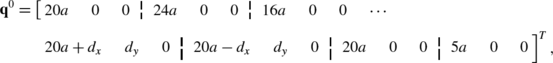(34)

where a=0.05 (m), d x =c x /2, d y =c y /2−b y , and quantities c x , c y , b y are defined in the Appendix.

It can be calculated that

$$\mathrm{rank}\bigl( \boldsymbol{\varPhi}_{\mathbf{q}}\bigl( \mathbf{q}^{0}\bigr) \bigr) = 17,\qquad \mathrm{rank}\bigl( \boldsymbol{\varPsi} \bigl(\mathbf{q}^{0}\bigr) \bigr) = 4,\qquad r = \mathrm{rank}\bigl( \mathbf{C}\bigl(\mathbf{q}^{0}\bigr) \bigr) = 20 < 17 + 4.$$
(35)

Matrix Φ q is a full-rank matrix, whereas Ψ is rank-deficient. Obviously, the constraint matrix C is rank-deficient as well. The same results can be obtained for any other nonsingular configuration q of the mechanism. This indicates that the considered multibody system is overconstrained. The degree of redundancy (see Eq. (7)) can be calculated as

$$p = m + h - r = 17 + 5 - 20 = 2.$$
(36)

Dependency of constraints was detected, thus the constraint reaction solvability analysis was performed prior to dynamic simulations. Firstly, QR decomposition of the constraint matrix C was calculated (see Eq. (21)). Then matrix C was divided into fourteen submatrices, corresponding to seven revolute joints, one translational joint, one driving constraint equation, and five knife-edge constraints, respectively. Similarly, matrix Q was divided into fourteen submatrices:

$$\mathbf{C}_{22 \times 21} = \left [ \begin{array}{c} {[} \mathbf{C}_{\mathrm{rev}A} ]_{2 \times 21} \\ \vdots\\ {[} \mathbf{C}_{\mathrm{rev}G} ]_{2 \times 21} \\ {[} \mathbf{C}_{\mathrm{tra}H} ]_{2 \times 21} \\ {[} \mathbf{C}_{\mathrm{drvng}} ]_{1 \times 21} \\ {[} \mathbf{C}_{\mathrm{ed}W_{1}} ]_{1 \times 18} \\ \vdots\\ {[} \mathbf{C}_{\mathrm{ed}W_{5}} ]_{1 \times 21} \\ \end{array} \right ],\qquad \mathbf{Q}_{22 \times 22} = \left [ \begin{array}{c} {[} \mathbf{Q}_{\mathrm{rev}A} ]_{2 \times 22} \\ \vdots\\ {[} \mathbf{Q}_{\mathrm{rev}G} ]_{2 \times 22} \\ {[} \mathbf{Q}_{\mathrm{tra}H} ]_{2 \times 22} \\ {[} \mathbf{Q}_{\mathrm{drvng}} ]_{1 \times 22} \\ {[} \mathbf{Q}_{\mathrm{ed}W_{1}} ]_{1 \times 22} \\ \vdots\\ {[} \mathbf{Q}_{\mathrm{ed}W_{5}} ]_{1 \times 22} \\ \end{array} \right ].$$
(37)

Next, Eq. (27) was utilized to calculate eleven matrices B X (note that degree of redundancy p=2, thus only two last columns of Q X matrices were used to build corresponding $$\mathbf{Q}_{X}^{\mathrm{col}}$$ matrices):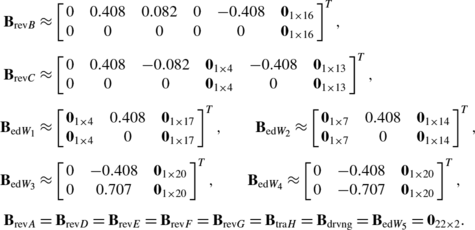(38)

Matrices corresponding to knife-edge kinematic pairs W 1W 4 as well as matrices corresponding to revolute joints B and C have nonzero elements, thus constraint reactions in these pairs cannot be uniquely determined using the rigid body model. Matrices corresponding to revolute joints A, D, E, F, and G, to translational joint H, and to the driving constraint equation consist of zeros only, thus related reactions can be uniquely determined. This concludes the constraint reactions solvability analysis.

### Simulated motion of the mechanism

Masses of mechanism bodies and their central moments of inertia are presented in Table 2. In each moving part, the center of its mass coincides with the local reference frame origin.

Equations of motion for the investigated mechanism can be written in the form of Eq. (11), with no external forces applied to the system (note that the edge-ground forces are modeled as constraint reactions), with M 21×21=diag(m 1,m 1,J 1,…,m 7,m 7,J 7), and with terms C and Γ discussed previously.

The mechanism is overconstrained, therefore, the leading matrix in the equations of motion is singular. The constraints elimination method (see Sect. 3.1) was chosen to handle the redundancy problem. Redundant constraints may be selected in many ways, thus several variants of dynamic simulations were carried out. In each simulation, the equations of motion were integrated (the fourth-fifth order Runge–Kutta formula , implemented in MATLAB® as ode45 function, was used) with the initial configuration given by q 0 (see Eq. (34)) and initial velocity $$\dot{\mathbf{q}}^{0}$$: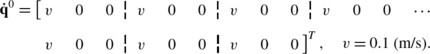(39)

Diverse joint reactions were calculated for different selections of redundant constraints, however, in each case the same mechanism motion (to within numerical precision) was obtained. This illustrates that—as it was discussed in Sect. 2.2—in the case of an overconstrained mechanism with frictionless kinematic pairs, the individual reactions are non-unique but their resultant effect (when motion is concerned) is unique.

The trajectory of robot platform center of mass as well as the trajectories of knife-edge contact points, observed during simulations, are presented in Fig. 3.

### Calculated constraint reactions

Three variants (out of several other possible) of redundant equations elimination were studied and presented here. Since the degree of redundancy equals two, in each possible variant two constraint equations need to be eliminated.

In the first variant, scalar constraint equations no. 6 and 21 were eliminated from the set of constraints at velocity level, i.e., from Eq. (4). Appropriate elimination of matrix C (see Eq. (33)) rows no. 6 and 21, as well as vector Γ elements no. 6 and 21, was performed. Consequently, the Lagrange multipliers associated with the neglected equations were canceled, and a reduced set of equations of motion (Eq. (11)), with nonsingular leading matrix, was obtained. Note that the eliminated constraint equation no. 6 represents revolute joint C, thus—as a result of the elimination process—the y component of joint C reaction was arbitrarily set to zero. Constraint equation no. 21 represents knife-edge kinematic pair W 4, thus reaction in this pair was set to zero as well.

In the second variant of redundant equations elimination, constraints no. 18 and 21, representing knife-edges W 1 and W 4, respectively, were eliminated. In the third variant, constraint equations no. 19 and 21, associated with knife-edges W 2 and W 4, respectively, were deleted. Again, appropriate reactions were set to zero due to purely mathematical operations, not supported by the physics of the system.

For all three variants of redundant constraints elimination, dynamic simulations were carried out and constraint reactions were observed (as it was mentioned earlier, simulated motion was the same in all cases). Reactions observed in joints A, D, E, F, G, H, the steering force associated with driving constraints, and knife-edge W 5 reaction had the same time histories in all simulations, whereas reactions in joints B and C, as well as knife-edges W 1W 4 reactions, were different in each case. The results of simulations corroborate correctness of constraint reactions solvability analysis performed in Sect. 4.2.

Global x and y components of revolute joint B reaction force and perpendicular to the edge component of the knife-edge W 3 reaction are presented in Fig. 4 as examples of nonunique reactions. In the same Fig. 4, components of revolute joint D reaction force and the knife-edge W 5 reaction are shown as examples of uniquely solvable reactions.

The alternatively eliminated constraint equations are associated with kinematic pairs C, W 1, W 2, and W 4. It is worth noting that in each case elimination affected, among others, reactions in pairs B and W 3. This result shows that the process of elimination influences not only reactions of neglected constraints but also reactions of constraints remaining in the mathematical model.

It should be mentioned that the redundant constraints elimination method can be seen as a substitution of the original mechanism by a kinematically equivalent mechanism without redundant constraints. The problem is that—when reactions are concerned—there is no equivalent model without redundant constraints. Elimination of a constraint results in setting its reaction to zero, which is unlikely to be observed in the original mechanism.

The presence of redundant constraints leads to nonuniqueness of some or all reactions, as long as the mechanism parts are treated as rigid bodies. Mathematically, redundant constraints existence results in system of equations with infinite number of solutions. Thus, the rigid body equations of motion are insufficient to calculate realistic joint reactions, observed in real-world overconstrained multibody systems.

Although the problem of reactions uniqueness—crucial for results credibility—is often encountered in practical calculations, surprisingly small attention is paid to it. In the great majority of general purpose multibody simulation packages detailed information whether particular joint reaction is solvable or unsolvable is unavailable, and the problem of reactions solvability is simply neglected. The software users are frequently advised to replace overconstrained models with kinematically equivalent models without redundant constraints. The problem that the nonredundant model does not reflect the real system is usually not discussed. If the software user fails to follow the advice, the redundant constraints detected in the model are handled automatically, using one of several available methods. This procedure may easily lead to erroneous results. For example, quite often the multibody software user is not aware that specified joint reaction force is nonunique and his attempt to introduce friction into this joint is pointless.

This paper presents a theoretical background and numerical methods for detecting kinematic pairs of rigid body mechanism for which reactions can be uniquely determined despite the redundancy of constraints. The reactions solvability analysis methods, previously proposed for holonomic systems, are generalized to mechanisms subjected to linear nonholonomic constraints. It should be emphasized that the obtained results are valid for both integrable and nonintegrable Pfaffian constraints. An example of a mobile robot analysis is provided to illustrate the discussed problems.

The proposed reactions solvability methods are based on constraint matrix investigation, thus they can be easily implemented in existing multibody software. Required computational effort is small, since the constraint matrix must be computed anyway. Moreover, in most cases, reactions solvability analysis can be performed only once, during the preprocessing phase of simulation (provided that the initial mechanism configuration is nonsingular), along with redundant constraint elimination process.

The uniqueness of reactions depends only upon the kinematic structure of the mechanism, thus it is irrelevant which type of coordinates is utilized to model the multibody system. The methods presented in the paper employ absolute coordinates’ formulation. Adopting them to use other types of coordinates, for example, relative ones, is not straightforward and requires additional research.

Finally, going beyond the scope of this paper, we should point out that in order to find unique values of all reactions, rigid body assumption must be rejected. Engineering experiences suggest that flexibility of bodies, elasticity of bearings, assembly stresses, thermal loads, joint clearances as well as geometric imperfections (in , it was shown that, in the case of redundantly constrained mechanisms, the rigid body assumption must be accompanied by a postulate that links are geometrically perfect) should be taken into account, when solving for realistic reactions. In many models, elasticity of bodies is considered, however, some effects associated with constraint redundancy are still observed, even when mechanisms are modeled as entirely flexible systems, e.g., [1, 21]. Quite often, in a multibody model, only selected rigid bodies are replaced by their deformable substitutes, e.g., , which not always leads to correct results. It can be shown that problems with finding realistic reactions, typical for overconstrained systems, may be observed when analyzing partially flexible models without redundant constraints . Hence, problems with finding reactions in overconstrained mechanisms are not limited to purely rigid body models.

## References

1. Aarts, R.G.K.M., Meijaard, J.P., Jonker, J.B.: Flexible multibody modelling for exact constraint design of compliant mechanisms. Multibody Syst. Dyn. 27(1), 119–133 (2012)

2. Bayo, E., Ledesma, R.: Augmented Lagrangian and mass-orthogonal projection methods for constrained multibody dynamics. Nonlinear Dyn. 9(1–2), 113–130 (1996)

3. Bayo, E., Garcia de Jalon, J., Serna, M.A.: A modified Lagrangian formulation for the dynamic analysis of constrained mechanical systems. Comput. Methods Appl. Mech. Eng. 71(2), 183–195 (1988)

4. Blajer, W.: On the determination of joint reactions in multibody mechanisms. J. Mech. Des. 126(2), 341–350 (2004)

5. Bloch, A.: Nonholonomic Mechanics and Control. Interdisciplinary Applied Mathematics, vol. 24. Springer, New York (2003)

6. Dormand, J.R., Prince, P.J.: A family of embedded Runge–Kutta formulae. J. Comput. Appl. Math. 6(1), 19–26 (1980)

7. Frączek, J., Wojtyra, M.: Teaching multibody dynamics at Warsaw University of Technology. Multibody Syst. Dyn. 13(3), 353–361 (2005)

8. Frączek, J., Wojtyra, M.: On the unique solvability of a direct dynamics problem for mechanisms with redundant constraints and Coulomb friction in joints. Mech. Mach. Theory 46(3), 312–334 (2011)

9. Frączek, J., Wojtyra, M.: Application of general multibody methods to robotics. In: Arczewski, K., et al. (eds.) Multibody Dynamics—Computational Methods and Applications, pp. 131–152. Springer, Berlin (2011)

10. Garcia de Jalon, J., Bayo, E.: Kinematic and Dynamic Simulation of Multibody Systems: The Real-Time Challenge. Springer, New York (1994)

11. Garcia de Jalon, J., Gutiérrez-López, M.D.: Multibody dynamics with redundant constraints and singular mass matrix. In: Proc. of the 2nd Joint International Conference on Multibody System Dynamics, Stuttgart, Germany (2012)

12. Golub, G.H., Van Loan, C.F.: Matrix Computations, 3rd edn. Johns Hopkins University Press, Baltimore (1996)

13. González, F., Kövecses, J.: Use of penalty formulations in the dynamic simulation of redundantly constrained multibody systems. In: Proc. of the 2nd Joint International Conference on Multibody System Dynamics, Stuttgart, Germany (2012)

14. González, F., Kövecses, J.: Use of penalty formulations in dynamic simulation and analysis of redundantly constrained multibody systems. Multibody Syst. Dyn. 29(1), 57–76 (2013)

15. González, F., Kövecses, J., Teichmann, M., Courchesne, M.: Techniques for the simulation of redundantly constrained multibody systems. In: Proc. of the ECCOMAS Thematic Conference Multibody Dynamics 2011, Brussels, Belgium (2011)

16. Haug, E.J.: Computer Aided Kinematics and Dynamics of Mechanical Systems. Allyn & Bacon, Boston (1989)

17. Kim, S.S., Vanderploeg, M.J.: QR decomposition for state space representation of constrained mechanical dynamic systems. J. Mech. Transm. Autom. Des. 108, 176–182 (1986)

18. Laulusa, A., Bauchau, O.A.: Review of classical approaches for constraint enforcement in multibody systems. J. Comput. Nonlinear Dyn. 3(1), 011005 (2008)

19. Malczyk, P., Frączek, J.: Cluster computing of mechanisms dynamics using recursive formulation. Multibody Syst. Dyn. 20(2), 177–196 (2008)

20. Malczyk, P., Frączek, J.: A divide and conquer algorithm for constrained multibody system dynamics based on augmented Lagrangian method with projections-based error correction. Nonlinear Dyn. 70(1), 871–889 (2012)

21. Meijaard, J.P., Brouwer, D.M., Jonker, J.B.: Analytical and experimental investigation of a parallel leaf spring guidance. Multibody Syst. Dyn. 23(1), 77–97 (2010)

22. Mianowski, K., Wojtyra, M.: Virtual prototype of a 6-DOF parallel robot. In: Proc. of Eleventh World Congress in Mechanism and Machine Science, Tianjin, P.R. China, pp. 1604–1608 (2004)

23. Müller, A.: A conservative elimination procedure for permanently redundant closure constraints in MBS-models with relative coordinates. Multibody Syst. Dyn. 16(4), 309–330 (2006)

24. Müller, A.: Generic mobility of rigid body mechanisms. Mech. Mach. Theory 44(6), 1240–1255 (2009)

25. Müller, A.: Semialgebraic regularization of kinematic loop constraints in multibody system models. J. Comput. Nonlinear Dyn. 6(4), 041010 (2011)

26. Neimark, J.I., Fufaev, N.A.: Dynamics of Nonholonomic Systems. Am. Math. Soc., Providence (1972)

27. Neto, M.A., Ambrósio, J.: Stabilization methods for the integration of DAE in the presence of redundant constraints. Multibody Syst. Dyn. 10(1), 81–105 (2003)

28. Nikravesh, P.E.: Computer-Aided Analysis of Mechanical Systems. Prentice Hall, Englewood Cliffs (1988)

29. Phong, D.V., Hoang, N.Q.: Singularity-free simulation of closed loop multibody systems by using null space of Jacobian matrix. Multibody Syst. Dyn. 27(4), 487–503 (2012)

30. Ruzzeh, B., Kövecses, J.: A penalty formulation for dynamics analysis of redundant mechanical systems. J. Comput. Nonlinear Dyn. 6(2), 021008 (2011)

31. Strang, G.: Linear Algebra and Its Applications, 4th edn. Brooks/Cole, Pacific Grove (2005)

32. Udwadia, F.E., Kalaba, R.E.: Analytical Dynamics: A New Approach. Cambridge University Press, New York (1996)

33. Wojtyra, M.: Joint reaction forces in multibody systems with redundant constraints. Multibody Syst. Dyn. 14(1), 23–46 (2005)

34. Wojtyra, M.: Joint reactions in rigid body mechanisms with dependent constraints. Mech. Mach. Theory 44(12), 2265–2278 (2009)

35. Wojtyra, M., Frączek, J.: Joint reactions in rigid or flexible body mechanisms with redundant constraints. Bull. Pol. Acad. Sci., Tech. Sci. 60(3), 617–626 (2012)

36. Wojtyra, M., Frączek, J.: Comparison of selected methods of handling redundant constraints in multibody systems simulations. J. Comput. Nonlinear Dyn. 8(2), 021007 (2013)

37. Zahariev, E., Cuadrado, J.: Dynamics of over-constrained rigid and flexible multibody systems. In: Proc. of the 12th IFToMM. World Congress, Besançon, France (2007)

## Acknowledgements

Research supported by the Institute of Aeronautics and Applied Mechanics statutory funds.

## Author information

Authors

### Corresponding author

Correspondence to Marek Wojtyra.

## Appendix: Holonomic constraints and their derivatives

### Appendix: Holonomic constraints and their derivatives

The investigated mechanism, presented in Fig. 1, consists of seven moving bodies interconnected by seven revolute joints and one translational joint. The joints are represented by geometric constraint equations. Moreover, driving constraints are imposed on relative motion in the translational joint.

Let us consider a revolute joint formed by bodies i and j at point P i =Q j (for example, in Fig. 1 joint D is formed by bodies 2 and 4, P 2=Q 4=D). The constraint equations describing this joint can be derived by requiring that the point P i on body i coincides with point Q j on body j:

$$\boldsymbol{\varPhi}^{r} = \mathbf{r}_{i} + \mathbf{R}_{i}\mathbf{s}_{P}^{(i)} - \mathbf{r}_{j} - \mathbf{R}_{j}\mathbf{s}_{Q}^{(j)} = \mathbf{0}_{2 \times 1},$$
(40)

where $$\mathbf{s}_{U}^{(k)}$$ is the position vector of point U k in the local reference frame π k and R k is the direction cosine matrix transforming quantities from π k to π 0:

$$\mathbf{R}_{k} = \mathbf{R}_{k}( \varphi_{k} ) = \left [ \begin{array}{c@{\quad }c} \cos\varphi_{k} & - \sin\varphi_{k} \\ \sin\varphi_{k} & \cos\varphi_{k} \\ \end{array} \right ].$$
(41)

Let us consider a translational joint, formed by bodies i and j, and assume that point P i belongs to body i, and point Q j as well as vector v ij belong to body j. Points P i and Q j lie on a line parallel to the axis of relative joint motion, and vector v ij is perpendicular to this axis. The translational joint can be described by two scalar constraint equations. The first one represents the fact that vector P i Q j is perpendicular to vector v ij , and the second equation represents the fact that body i does not change its orientation with respect to body j:

$$\boldsymbol{\varPhi}^{t} = \left [ \begin{array}{c} ( \mathbf{r}_{j} + \mathbf{R}_{j}\mathbf{s}_{Q}^{(j)} - \mathbf{r}_{i} - \mathbf{R}_{i}\mathbf{s}_{P}^{(i)} )^{T}\mathbf{R}_{j}\mathbf{v}_{ij}^{(j)} \\ \varphi_{i} - \varphi_{j} - \psi_{ij} \\ \end{array} \right ] = \mathbf{0}_{2 \times 1},$$
(42)

where ψ ij is a constant angular value.

The driving constraint (single scalar equation) for a translational joint can be derived by requiring that the distance between points P i and Q j , at every time instant, is given by a scalar, time-dependent function d(t):

$$\varPhi^{d} = \bigl( \mathbf{r}_{j} + \mathbf{R}_{j}\mathbf{s}_{Q}^{(j)} - \mathbf{r}_{i} - \mathbf{R}_{i}\mathbf{s}_{P}^{(i)} \bigr)^{T}\mathbf{R}_{j}\mathbf{u}_{ij}^{(j)} - d(t) = 0,$$
(43)

where u ij is a unit vector parallel to the line of relative motion.

Geometric parameters characterizing joints are presented in Table 3. Note that a=0.05 (m), and BD=CE=a, DF=EG=5a, BC=LM=8a, AC=4a, AN=7a (see Fig. 1).

The nonzero Jacobian matrix entries (see Eq. (2)) corresponding to Eq. (40) can be calculated as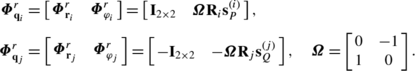(44)

The nonzero Jacobian matrix entries corresponding to Eq. (42) are the following: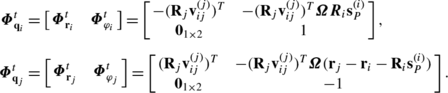(45)

The nonzero Jacobian matrix entries corresponding to Eq. (43), and the nonzero elements of the driving constraints partial derivative with respect to time (see Eq. (2)), are the following: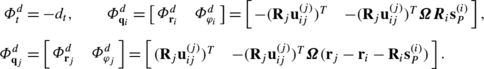(46)

Vectors Γ (see Eq. (9)), representing revolute and translational joints as well as the driving constraints, can be respectively expressed as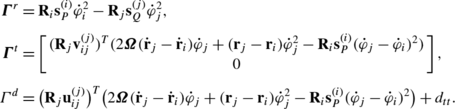(47)

## Rights and permissions

Reprints and Permissions

Wojtyra, M., Frączek, J. Solvability of reactions in rigid multibody systems with redundant nonholonomic constraints. Multibody Syst Dyn 30, 153–171 (2013). https://doi.org/10.1007/s11044-013-9352-0

• Accepted:

• Published:

• Issue Date:

• DOI: https://doi.org/10.1007/s11044-013-9352-0

### Keywords

• Redundant constraints
• Nonholonomic systems
• Constraint reactions